# Oxalic acid, H2C2O4*2H2O (molar mass = 126.07 g/mol) is oftenused as a primary standard

Oxalic acid, H2C2O4*2H2O (molar mass = 126.07 g/mol) is oftenused as a primary standard for the standardization of a NaOHsolution. If 0.147 g of oxalic acid dihydrate is nutralizedby 23.64mL of a NaOH solution, what is the molar concentration ofthe NAOH solution? Oxalic acid is a diprotic acid. (What is thebalanced equation?)

Concepts and reason
Law of conservation of mass states that, in a chemical reaction, mass is neither created nor destroyed. Thebalanced chemical equation can be obtained using this phenomenon.Mass and molar concentration can be calculated by using this balanced chemical equation.

Fundamentals
Law of conservation of mass: mass is neither created nor destroyed in a chemical reaction which states that the mass of the reactants before the reaction is equal to the mass of the products after the reaction.
Balanced chemical equation: this is an application for the law of conservation of mass; which states that the number of atoms presents on the reactant side is equal to the atoms present in the product side.
**Molar mass:**The mass of one mole of compound is called molar mass.
Molarity: The number of moles of solute present in unit volume of solution.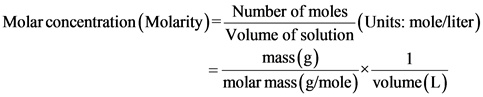Given reaction statement
Oxalic acid is often used as a primary standard for the standardization for NaOH.
The balanced chemical equation is given below: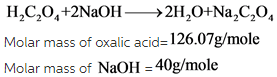According to the balanced chemical equation, it is clear that 126.07g of oxalic acid required for standardizing the 80 grams of NaOH
Given that
0.147g of oxalic acid is used as a primary standard for standardizing the NaOH
Let required weight of NaOH for standardizing be ‘X’.
Therefore,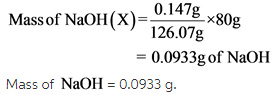The balanced chemical equation is written by using the given reaction statement and calculated the mass NaOH of required for the 0.147g of oxalic acid, which is used as a primary standard in the standardization of NaOH.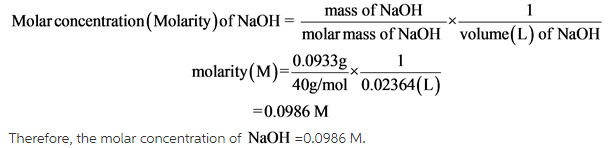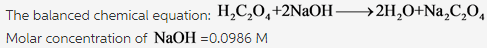Molar concentration (molarity) of NaOH is calculated by finding the moles NaOH of and dividing it with volume of solution.